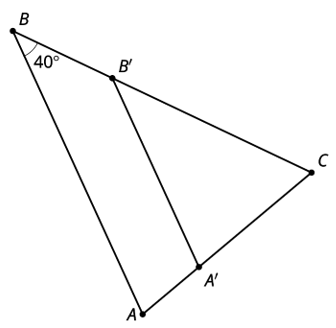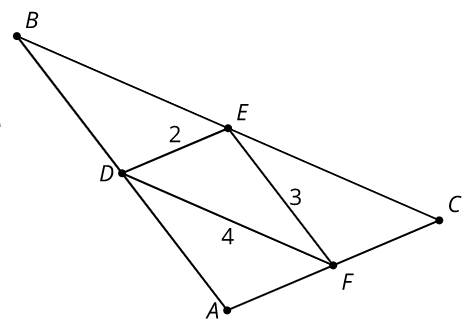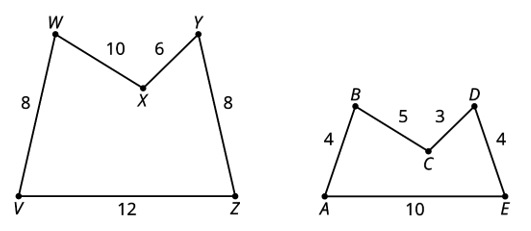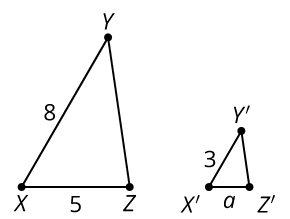# G.3.5 Practice Problems

What is the measure of angle A'B'C?Check all that apply

Triangle DEF is formed by connecting the midpoints of the sides of triangle ABC. The lengths of the sides of DEF are shown. What is the length of AB?Angle ABC is taken by a dilation with center P and scale factor 1/3 to angle A'B'C'. The measure of angle ABC is 21°. What is the measure of angle A'B'C'?

Draw 2 lines that could be the image of line m by a dilation.

Is it possible for polygon ABCDE to be dilated to figure VWXYZ? Explain your reasoning.Triangle XYZ is scaled and the image is X'Y'Z'. Write 2 equations that could be used to solve for a.